# 一.任务代码:

#include <stdio.h>
#include <stdlib.h>
#include <string.h>
struct student {
char name1;
char name2;
char num;
char sex;
int score;
};
struct student students;
void Space(int num);
void write();
int main() {
system("color f4");
printf("   Welcome to this wonderful world!    \n");
while (1)
{
Space(20);
printf("******************请输入符号选择你所需要的功能*******************\n");
Space(20);
printf("|                            1.WRITE                             |\n");
Space(20);
Space(20);
printf("|                             3.EXIT                             |\n");
Space(20);
printf("*****************************************************************\n");
int choice;
scanf("%d", &choice);
switch (choice) {
case 1:write();
break;
break;
case 3:printf("Thanks!\n");
return 0;
break;
}
system("pause");//冻结窗口
system("cls");//清屏
}
return 0;
}

void write() {
FILE* fp;
char name2;
int i = 0;
printf("姓名:\n");
scanf("%s", students[i].name2);
strcpy(students[i].name1, students[i].name2);
strcat(students[i].name1, ".txt");
if ((fp = fopen(students[i].name1, "w")) == NULL)//判断是否正常打开文件
{
printf("Can not open the file!\n");
exit(0);
}
printf("学号:\n");
scanf("       %s", students[i].num);
printf("性别:\n");
scanf("       %s", students[i].sex);
printf("班级:\n");
printf("线代成绩：\n");
scanf("           %d", &students[i].score);
fp = fopen(students[i].name1, "a+");
fprintf(fp, "%s    %s     %s     %d\n", students[i].num, students[i].sex, students[i].grade, students[i].score);
if (fclose(fp)) {             //判断是否能正常关闭文件
printf("Can not close the file！\n");
exit(0);
}
}
{
FILE* fp;
int j = 0;
char name1, name2;
printf("姓名：\n");
scanf("%s", name1);
strcpy(name2, name1);
strcat(name1, ".txt");
if ((fp = fopen(name1, "r")) == NULL)
{
printf("Can not open the file!\n");
exit(0);
}
strcpy(name1, name2);
strcat(name1, ".txt");
fp = fopen(name1, "r");
fscanf(fp, "%s    %s    %s    %d", students[j].num, students[j].sex, students[j].grade, &students[j].score);
printf("姓名：%s   学号：%s   性别：%s   班级：%s   线代成绩：%d\n", name2, students[j].num, students[j].sex, students[j].grade, students[j].score);
fclose(fp);           //关闭文件
system("pause");     //冻结窗口
}

void Space(int num) {
for (int i = 0; i < num; i++) {
printf(" ");
}

}


# 二.运行后的截图：

## 1.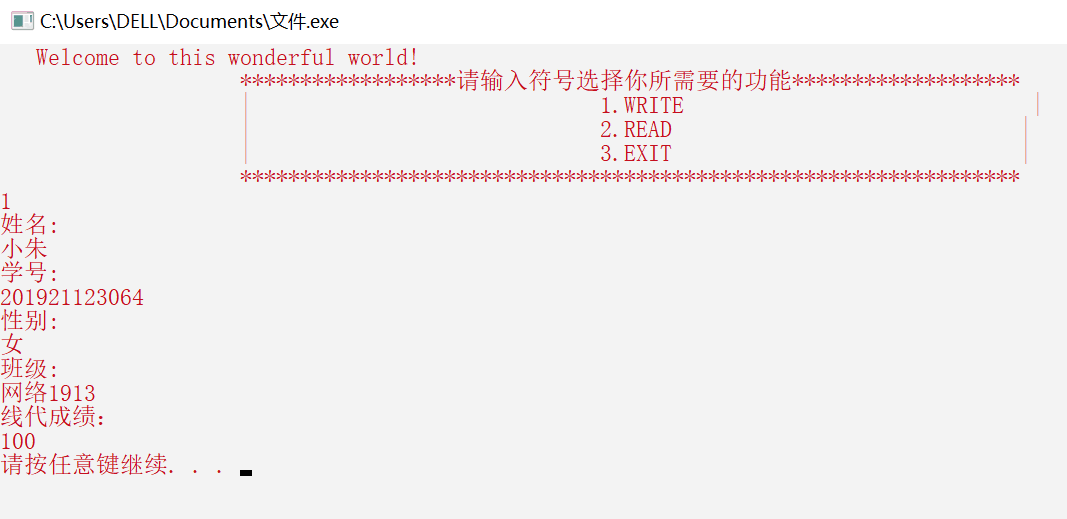### 2.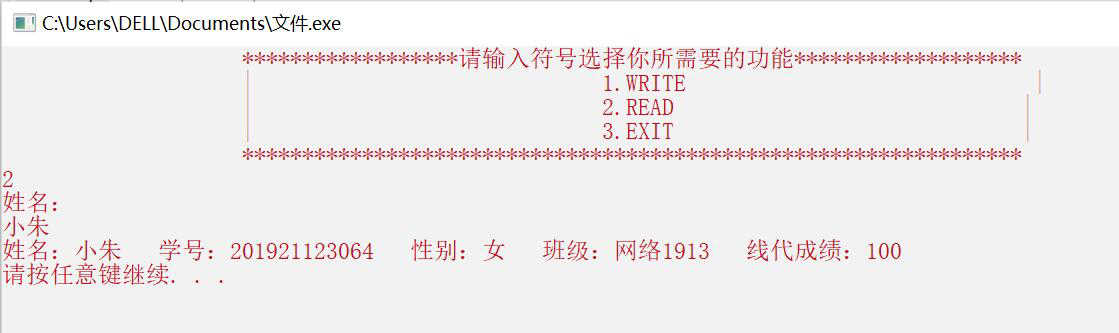## 3.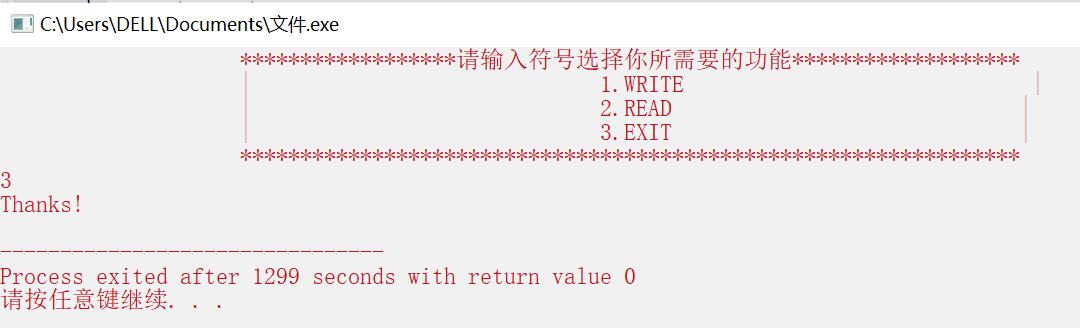## 4.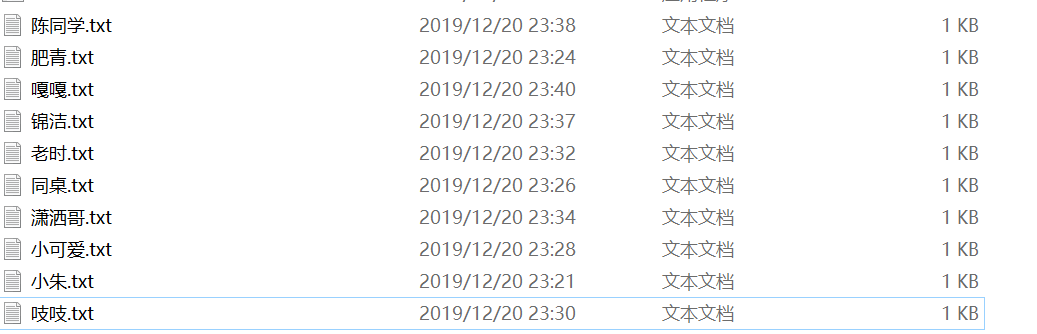## 5.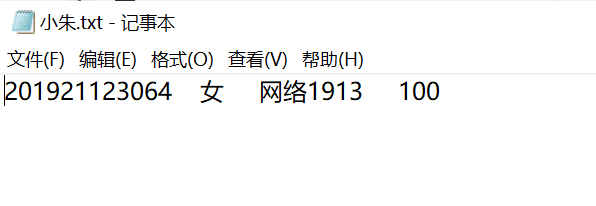# 二.知识点：

1.全缓冲

2.行缓冲

3.不带缓冲

## 3.system:

1.system是一个函数，用于运行其它外部程序。函数原型：int system(const char * string);
2.system("cls"),是执行一个CMD中的命令cls，这是清屏命令;
3.system("PAUSE") 是暂停的意思，等待用户信号；不然控制台程序会一闪即过，你来不及看到执行结果;
4.system的背景美化：

0 = 黑色 8 = 灰色
1 = 蓝色 9 = 淡蓝色
2 = 绿色 A = 淡绿色
3 = 浅绿色 B = 淡浅绿色
4 = 红色 C = 淡红色
5 = 紫色 D = 淡紫色
6 = 黄色 E = 淡黄色
7 = 白色 F = 亮白色

# 三.我的总结：

1.我终于会把我的输出界面打扮得漂漂亮亮了，学会了用sytem的基本操作，及冻结、清屏、美化；
2.会用“fopen,fclose"读取和关闭文件，还有用“fscanf,fprintf"读取和写入数据；

posted @ 2019-12-21 14:11    阅读(157)  评论(0编辑  收藏  举报